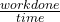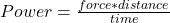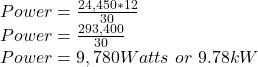## PLS ANSWER WILL GIVE BRAINLIEST An elevator with a person inside weighs a total of 24,450 Newtons. an elevator that rises 12 meters in

Question

An elevator with a person inside weighs a total of 24,450 Newtons. an elevator that rises 12 meters in 30 seconds. How much power is needed for the elevator trip?

in progress 0
6 months 2021-09-04T08:33:31+00:00 2 Answers 1 views 0

9780 W

Explanation:

Power: This can be defined as the rate at which work is done. The S.I unit of power is Watt (W).

From the question,

P = W/t………………….. Equation 1

Where P = power, W = work, t = time.

But,

W = F×d………….. Equation 2

Where F = force, d = distance.

Substitute equation 2 into equation 1

P = (F×d)/t…………….. Equation 3

Given: F = 24450 N, d = 12 m, t = 30 s.

Substitute these vales into equation 3

P = (24450×12)/30

P = 9780 W.

Hence the power needed for the elevator trip = 9780 W

## 9,780Watts

Explanation:

Power is defined as the rate of workdone with respect to time.

The formula for calculating power P needed by the eleveator is expressed as;

Power =Since Workdone = Force*distance then;Given the weight of elevator = 24,450Newtons

distance moved by the elevator = 12m

time taken = 12seconds.

Substituting this values in the formula given will give;The amount of power needed for the elevator trip is 9,780Watts